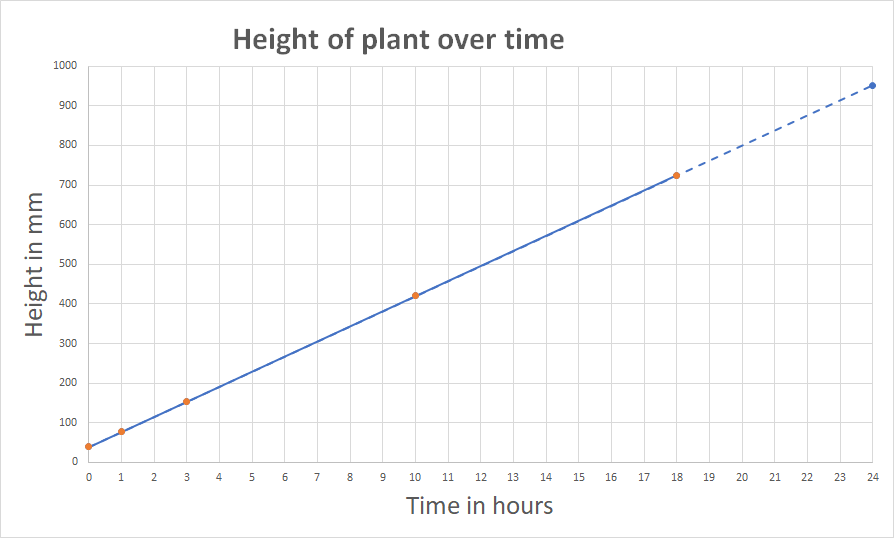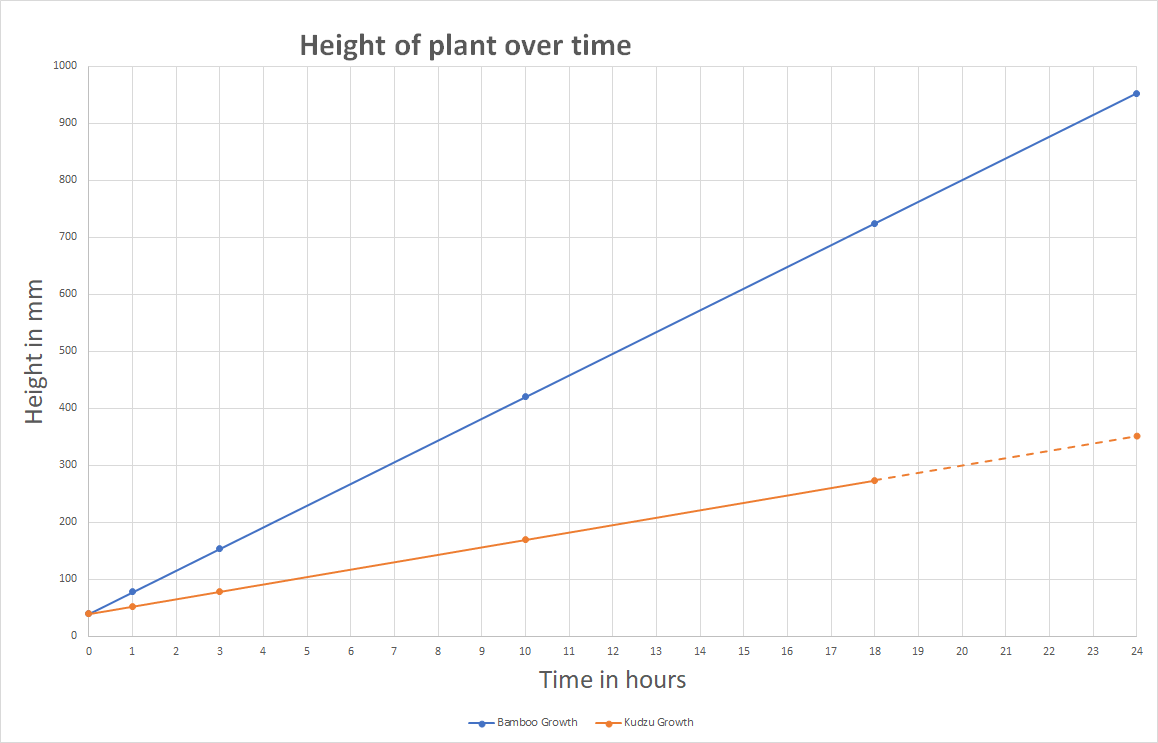Back
Interpreting Slope

The slope of a line represents how the y-axis values change compared to the numbers on the x-axis. This is also called the rate of change.

• Positive slope = y-values are growing compared to the x-values
• Negative slope = y-values are decreasing/decaying compared to the x-values
• 0-slope = there is no change in y-values compared to the x-values

We have also seen the following:

• The larger the slope = the steeper the line, or in other words, the greater the rate of change.
• The smaller the slope = the slower the growth or decay and slower the rate of change.
• The rate of change tells us how much change there is over a single unit of measure. It can also help us forecast what will happen in the future.

### Example:

The following table has the data for the height of a plant at different times. Note that the plant is already 40 mm tall when the data starts to be collected.

Just looking at this data, it is hard to visualize what the data is telling us, but a graph can help us. In this case, the independent variable (x-axis) is time because time happens whether the plant grows or not. It is not dependent on the height of the plant. The y-axis is the dependent variable because the height of the plant depends on how long it has been growing.

X-axis -- Time in Hours Y-axis -- Height in mm
0 40
1 78
3 154
10 420
18 724

Simply looking at this data, it is hard to know what it means. Does this mean the plant is growing quickly, or slowly, or not at all. A graph can help us visualize this.

Here is the data on a graph. We can use the data and the graph to calculate the slope of the line. This slope tells us how much the plant grows on average each hour. In other words, the slope is the rate of change, or in this case, the rate of growth.

## Find the slope

### 1. Stair step method

We see the growth of the line between x=0 and x=1 is the growth over one unit on the x-axis. We have the y-values for these points from the data points so we can use them to calculate the rise of this stair step.

Below is a portion of the graph zoomed in between x=0 and x=1.

It is important to note that the scale of the x-axis numbers is different than the scale of the y-axis numbers. This is why the amount of change in the x direction is 1 and the amount of change in the y direction is 38. This means the slope is 38.

### 2. Another method is to use the slope formula:

$${\text{Slope}}={\text{m}}=\dfrac{\text{Rise}}{\text{Run}}=\dfrac{\text{Change in y values}}{\text{Change in x values}}$$

This information tells us that within one hour, the plant grows 38 mm. This is the slope. Since our line is a straight line, the slope for the rest of the line is the same. We can use this to answer some of the following types of questions including forecasting what will happen in the future.

### Practice Problems

Use the previous information from this lesson about the growth of a plant to answer each of the following questions:
1. Assuming the growth remains the same, how much will the plant grow in the next hour? (
Solution
Solution: 38 mm
Details:
This question is again asking for the rate of change or slope. In this case it will grow an additional 38 mm.
)
2. Assuming the growth remains the same, how tall will the plant be at the end of the next hour? (
Solution
Solution: 762 mm at 19 hours
Details:
This question is asking for us to use the rate of change and add it to the last y-value of the data.
$$724 + 38 = 762\; \text{mm}$$

The plant will be 762 mm at 19 hours.
)
3. How tall will the plant be at the end of one day (24 hours)? (
Solution
Solution: $$724+228=952\;\text{mm}$$ tall
Details:
We can use the slope to help us forecast the graph. The first way to do this is to extend the line out to when $$x=24$$ to estimate the point. A more accurate way to forecast is to we add the rate of change for every additional hour needed up to 24 hours.

In this case, our last height measurement was at 18 hours and was 724 mm. If the plant grows at this same rate for 6 more hours it will grow $$6\times38=228\; \text{mm}$$ more. Thus, at the end of one day (24 hours) it will be $$724+228=952\;\text{mm}$$ tall.)

The plant we have been talking about in this example is bamboo. It is one of the fastest growing plants in the world and can grow up to 912 mm a day, close to three feet per day.

We can see this is significant by comparing the rate of change of the bamboo plant to the rate of change of kudzu, another fast growing plant. The data for the kudzu are shown in this table.

X-axis -- Time in Hours Height in mm of Bamboo Height in mm of Kudzu
0 40 40
1 78 53
3 154 79
10 420 170
18 724 274
24 952

Here is a graph that shows the line for the growth of bamboo (blue line) and the line for kudzu (orange line).

By looking at the data on the graph we can see the rate of change of bamboo is much greater than the rate of change of kudzu even though both grow quickly. We can use the graph for kudzu to also answer many of the same questions as we answered for bamboo, but first we must find the slope for kudzu’s growth.

This time we are using the stair step method, but our step is not one unit long. Instead it is 8 units long. In order to find the rate of change for the step we divide the rise by the run.

$$\dfrac{\text{rise}}{\text{run}}=\dfrac{104}{8}=\dfrac{13}{1}=13$$
This means the rate of change for kudzu is 13 mm/hour.

We can use this to now answer the questions for kudzu.

### Practice Problems

Use the previous information from this lesson about the growth of kudzu to answer each of the following questions:

4. Assuming the growth of the kudzu remains the same, how much will the plant grow in the next hour? (
Solution
Solution: 13 mm
Details:
This is the rate of change. The answer is 13mm in the next hour.
)
5. Assuming the growth of kudzu remains the same, how tall will the plant be at the end of the next hour? (
Solution
Solution: $$274+13=287\; \text{mm}$$
Details:
Our last data point was at 18 hours, so this wants to know how tall it will be at 19 hours. If it continues to grow an additional 13 mm each hour, it will be $$274+13=287\; \text{mm}$$.
)
6. How tall will the kudzu be at the end of one day (24 hours)? (
Solution
Solution: $$274+78=352\; \text{mm}$$
Details:
Our last data point was at 18 hours, so this wants to know how tall it will be 6 hours later.
In 6 hours the kudzu will grow $$6x\times13=78\; \text{mm}$$.

This means the total height at 24 hours will be $$274+78=352\; \text{mm}$$.
If we extend the line out to 24 hours we can see this on the graph.)

## Need More Help?

1. Study other Math Lessons in the Resource Center.
2. Visit the Online Tutoring Resources in the Resource Center.